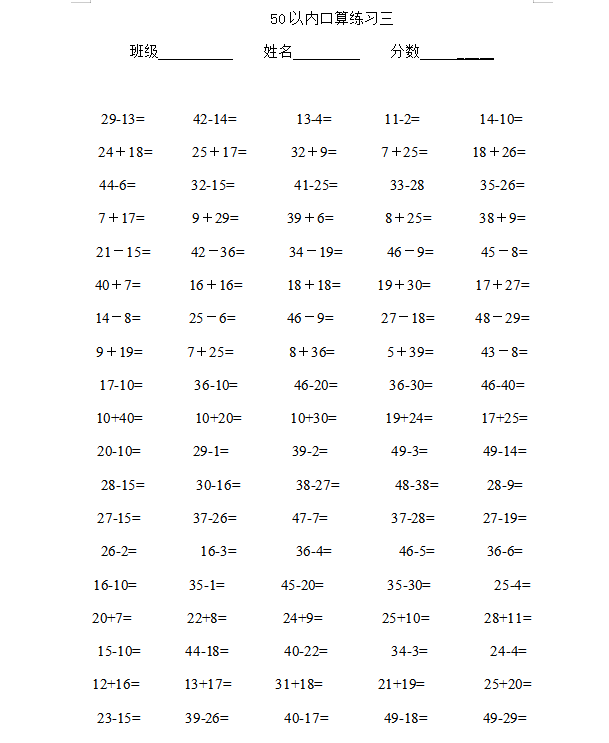50以内口算练习三

29-13=          42-14=            13-4=          11-2=           14-10=
24＋18=          25＋17=          32＋9=         7＋25=         18＋26=
44-6=           32-15=            41-25=           33-28           35-26=
7＋17=           9＋29=           39＋6=           8＋25=          38＋9=
21－15=         42－36=          34－19=          46－9=         45－8=
40＋7=           16＋16=          18＋18=         19＋30=         17＋27=